# Maths important formula - BINOMIAL THEOREM

BINOMIAL THEOREM

1.Statement of Binomial Theorem:

if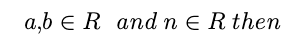2. Properties of Binomial Theorem:

i. General term :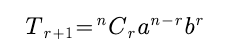ii. middle term(s):

a. if n is even, there is only one middle term which is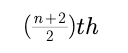term.

b. if n is odd, there are two middle terms which are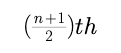and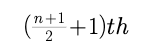term

3.Multinomial Theorem :Here total number of terms in the expansions =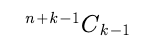4. Application of Binomial Theorem:

if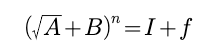where I and n are positive intergers, n beign odd and 0 < f <1 then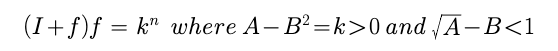if n is an even integer, then (I+f)(I - f )= k^n

5. Properties of Binomial Coefficients :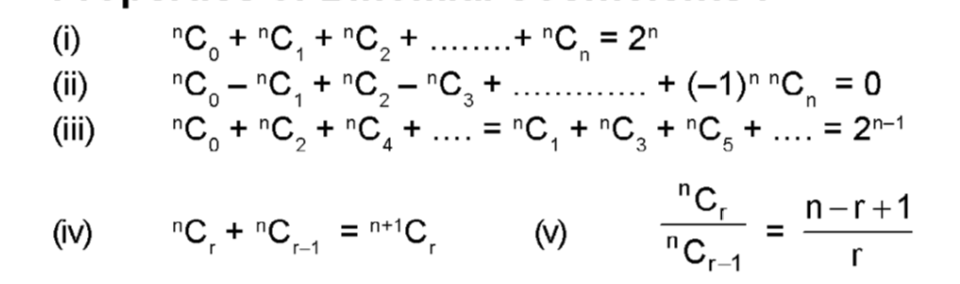Post By : Preeti Rai 13 Apr, 2020 556 views Maths